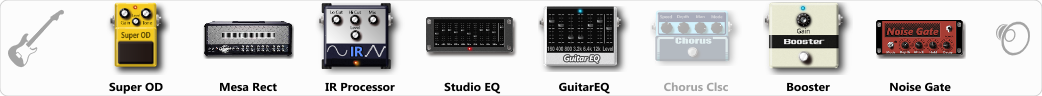# IR Tone 01 JD

Discussion in 'ToneLib-GFX presets' started by Joel Dreaver, Feb 7, 2020.

1. IR Tone 01 JD

Preset name: IR Tone 01 JD

Effects chain:Effect: "Super OD" (Overdrive / Distortion), active - "yes"
"Drive" = 33
"Tone" = 77
"Level" = 95

Effect: "Mesa Rect" (Amp simulators), active - "yes"
"Gain" = 61
"Bass" = 35
"Middle" = 38
"Treble" = 77
"Presence" = 56
"Master" = 50
"Output" = 50
"Level (dB)" = 7

Effect: "IR Processor" (Dynamics / Filter), active - "yes"
"IR" = PanteraVulgarDisplayOfPower
"Low Cut (Hz)" = 17
"Hi Cut (kHz)" = 13.0
"Mix" = 100
"Level (dB)" = 0

Effect: "Studio EQ" (Dynamics / Filter), active - "yes"
"31 Hz" = -1
"62 Hz" = -2
"125 Hz" = 1
"250 Hz" = -1
"500 Hz" = -7
"1 kHz" = -1
"2 kHz" = -2
"4 kHz" = 1
"8 kHz" = 6
"16 kHz" = 0
"above 16 kHz" = 0
"Level (dB)" = 0

Effect: "GuitarEQ" (Dynamics / Filter), active - "yes"
"160 Hz" = 0
"400 Hz" = 4
"800 Hz" = 4
"1.6 kHz" = 4
"3.2 kHz" = -1
"6.4 kHz" = 5
"12 kHz" = -2
"Level (dB)" = 0

Effect: "Chorus Clsc" (Modulation / Sfx), active - "no"
"Speed" = 4.8
"Depth" = 53
"Center" = 1.0
"Mode" = Stereo

Effect: "Booster" (Dynamics / Filter), active - "yes"
"Gain" = 64

Effect: "Noise Gate" (Dynamics / Filter), active - "yes"
"Detect" = Gtr In
"Mode" = Manual
"Depth" = 94
"Threshold" = 64
"Attack" = 0
"Hold" = 21
"Decay" = 282

Note: You will need to download and install the ToneLib-GFX software to use the preset.

#### Attached Files:

• ###### IR_Tone_01_JD.tlgfx
File size:
3.5 KB
Views:
2,983
Burzoom likes this.